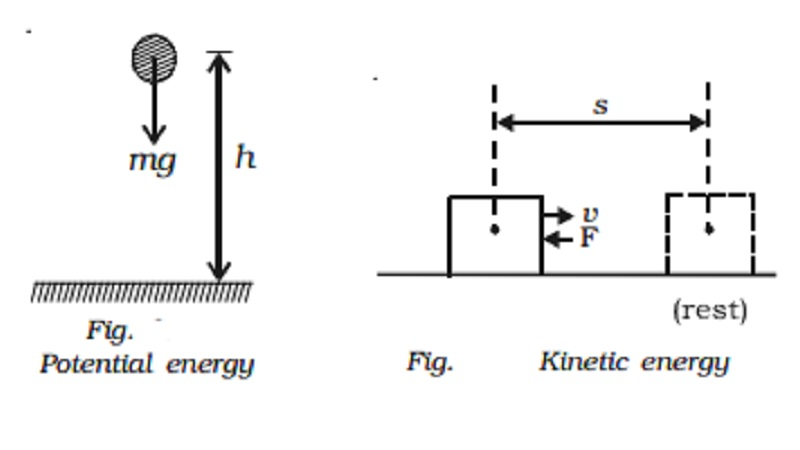Home | | Physics | | Physics | Potential energy and Kinetic energy

# Potential energy and Kinetic energyEnergy can be defined as the capacity to do work. Energy can manifest itself in many forms like mechanical energy, thermal energy, electric energy, chemical energy, light energy, nuclear energy, etc.

Energy

Energy can be defined as the capacity to do work. Energy can manifest itself in many forms like mechanical energy, thermal energy, electric energy, chemical energy, light energy, nuclear energy, etc.

The energy possessed by a body due to its position or due to its motion is called mechanical energy.

The mechanical energy of a body consists of potential energy and kinetic energy.

Potential energy

The potential energy of a body is the energy stored in the body by virtue of its position or the state of strain. Hence water stored in areservoir, a wound spring, compressed air, stretched rubber chord, etc, possess potential energy.

Potential energy is given by the amount of work done by the force acting on the body, when the body moves from its given position to some other position.Expression for the potential energy

Let us consider a body of mass m, which is at rest at a height h above the ground as shown in Fig. The work done in raising the body from the ground to the height h is stored in the body as its potential energy and when the body falls to the ground, the same amount of work can be got back from it. Now, in order to lift the body vertically up, a force mg equal to the weight of the body should be applied.

When the body is taken vertically up through a height h, then work done, W = Force x displacement

W = mg x h

This work done is stored as potential energy in the body

EP = mgh

Kinetic energy

The kinetic energy of a body is the energy possessed by the body by virtue of its motion. It is measured by the amount of work that the body can perform against the impressed forces before it comes to rest. A falling body, a bullet fired from a rifle, a swinging pendulum, etc. possess kinetic energy.

A body is capable of doing work if it moves, but in the process of doing work its velocity gradually decreases. The amount of work that can be done depends both on the magnitude of the velocity and the mass of the body. A heavy bullet will penetrate a wooden plank deeper than a light bullet of equal size moving with equal velocity.Expression for Kinetic energy

Let us consider a body of mass m moving with a velocity v in a straightline as shown in Fig.. Suppose that it is acted upon by a constant force F resisting its motion, which produces retardation (decrease in acceleration is known as retardation). Then

F = mass x retardation = - ma   ......(1)

Let dx be the displacement of the body before it comes to rest.

But the retardation isThis work done is equal to kinetic energy of the body.

Kinetic energy Ek = 1/2 mv2

Study Material, Lecturing Notes, Assignment, Reference, Wiki description explanation, brief detail

Related Topics